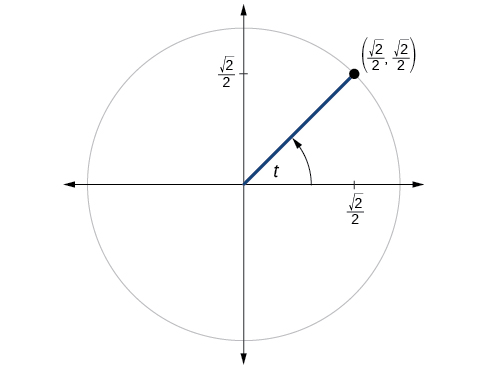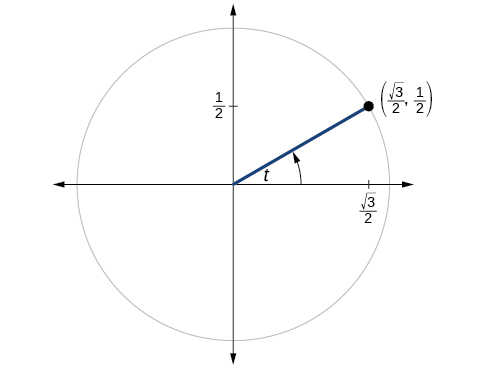# 7.4 The other trigonometric functions  (Page 7/14)

 Page 7 / 14

If $\mathrm{tan}\text{\hspace{0.17em}}t=\frac{12}{5},$ and $\text{\hspace{0.17em}}0\le t<\frac{\pi }{2},$ find $\text{\hspace{0.17em}}\mathrm{sin}\text{\hspace{0.17em}}t,\mathrm{cos}\text{\hspace{0.17em}}t,\mathrm{sec}\text{\hspace{0.17em}}t,\mathrm{csc}\text{\hspace{0.17em}}t,\text{and}\text{\hspace{0.17em}}\mathrm{cot}\text{\hspace{0.17em}}t.$

If $\text{\hspace{0.17em}}\mathrm{sin}\text{\hspace{0.17em}}t=\frac{\sqrt{3}}{2}\text{\hspace{0.17em}}$ and $\text{\hspace{0.17em}}\mathrm{cos}\text{\hspace{0.17em}}t=\frac{1}{2},$ find $\text{\hspace{0.17em}}\mathrm{sec}\text{\hspace{0.17em}}t,\mathrm{csc}\text{\hspace{0.17em}}t,\mathrm{tan}\text{\hspace{0.17em}}t,$ and $\text{\hspace{0.17em}}\mathrm{cot}\text{\hspace{0.17em}}t.$

$\mathrm{sec}t=2,$ $\mathrm{cot}t=\frac{\sqrt{3}}{3}$

If $\text{\hspace{0.17em}}\mathrm{sin}\text{\hspace{0.17em}}40°\approx 0.643\text{\hspace{0.17em}}$ and $\text{\hspace{0.17em}}\mathrm{cos}\text{\hspace{0.17em}}40°\approx 0.766,$ find $\text{\hspace{0.17em}}\text{sec}\text{\hspace{0.17em}}40°,\text{csc}\text{\hspace{0.17em}}40°,\text{tan}\text{\hspace{0.17em}}40°,$ and $\text{\hspace{0.17em}}\text{cot}\text{\hspace{0.17em}}40°.$

If $\text{\hspace{0.17em}}\text{sin}\text{\hspace{0.17em}}t=\frac{\sqrt{2}}{2},$ what is the $\text{\hspace{0.17em}}\text{sin}\left(-t\right)?$

$-\frac{\sqrt{2}}{2}$

If $\text{\hspace{0.17em}}\text{cos}\text{\hspace{0.17em}}t=\frac{1}{2},$ what is the $\text{\hspace{0.17em}}\text{cos}\left(-t\right)?$

If $\text{\hspace{0.17em}}\text{sec}\text{\hspace{0.17em}}t=3.1,$ what is the $\text{\hspace{0.17em}}\text{sec}\left(-t\right)?$

3.1

If $\text{\hspace{0.17em}}\text{csc}\text{\hspace{0.17em}}t=0.34,$ what is the $\text{\hspace{0.17em}}\text{csc}\left(-t\right)?$

If $\text{\hspace{0.17em}}\text{tan}\text{\hspace{0.17em}}t=-1.4,$ what is the $\text{\hspace{0.17em}}\text{tan}\left(-t\right)?$

1.4

If $\text{\hspace{0.17em}}\text{cot}\text{\hspace{0.17em}}t=9.23,$ what is the $\text{\hspace{0.17em}}\text{cot}\left(-t\right)?$

## Graphical

For the following exercises, use the angle in the unit circle to find the value of the each of the six trigonometric functions.$\mathrm{sin}t=\frac{\sqrt{2}}{2},\mathrm{cos}t=\frac{\sqrt{2}}{2},\mathrm{tan}t=1,\mathrm{cot}t=1,\mathrm{sec}t=\sqrt{2},\mathrm{csc}t=\sqrt{2}$$\mathrm{sin}t=-\frac{\sqrt{3}}{2},\mathrm{cos}t=-\frac{1}{2},\mathrm{tan}t=\sqrt{3},\mathrm{cot}t=\frac{\sqrt{3}}{3},\mathrm{sec}t=-2,\mathrm{csc}t=-\frac{2\sqrt{3}}{3}$

## Technology

For the following exercises, use a graphing calculator to evaluate to three decimal places.

$\mathrm{csc}\text{\hspace{0.17em}}\frac{5\pi }{9}$

$\mathrm{cot}\text{\hspace{0.17em}}\frac{4\pi }{7}$

–0.228

$\mathrm{sec}\text{\hspace{0.17em}}\frac{\pi }{10}$

$\mathrm{tan}\text{\hspace{0.17em}}\frac{5\pi }{8}$

–2.414

$\mathrm{sec}\text{\hspace{0.17em}}\frac{3\pi }{4}$

$\mathrm{csc}\text{\hspace{0.17em}}\frac{\pi }{4}$

1.414

$\text{tan}\text{\hspace{0.17em}}98°$

$\mathrm{cot}\text{\hspace{0.17em}}33°$

1.540

$\mathrm{cot}\text{\hspace{0.17em}}140°$

$\mathrm{sec}\text{\hspace{0.17em}}310°$

1.556

## Extensions

For the following exercises, use identities to evaluate the expression.

If $\text{\hspace{0.17em}}\mathrm{tan}\left(t\right)\approx 2.7,$ and $\text{\hspace{0.17em}}\mathrm{sin}\left(t\right)\approx 0.94,$ find $\text{\hspace{0.17em}}\mathrm{cos}\left(t\right).$

If $\text{\hspace{0.17em}}\mathrm{tan}\left(t\right)\approx 1.3,$ and $\text{\hspace{0.17em}}\mathrm{cos}\left(t\right)\approx 0.61,$ find $\text{\hspace{0.17em}}\mathrm{sin}\left(t\right).$

$\mathrm{sin}\left(t\right)\approx 0.79$

If $\text{\hspace{0.17em}}\mathrm{csc}\left(t\right)\approx 3.2,$ and $\text{\hspace{0.17em}}\mathrm{cos}\left(t\right)\approx 0.95,$ find $\text{\hspace{0.17em}}\mathrm{tan}\left(t\right).$

If $\text{\hspace{0.17em}}\mathrm{cot}\left(t\right)\approx 0.58,$ and $\text{\hspace{0.17em}}\mathrm{cos}\left(t\right)\approx 0.5,$ find $\text{\hspace{0.17em}}\mathrm{csc}\left(t\right).$

$\mathrm{csc}t\approx 1.16$

Determine whether the function $\text{\hspace{0.17em}}f\left(x\right)=2\mathrm{sin}x\text{\hspace{0.17em}}\mathrm{cos}\text{\hspace{0.17em}}x\text{\hspace{0.17em}}$ is even, odd, or neither.

Determine whether the function $\text{\hspace{0.17em}}f\left(x\right)=3{\mathrm{sin}}^{2}x\text{\hspace{0.17em}}\mathrm{cos}\text{\hspace{0.17em}}x+\mathrm{sec}\text{\hspace{0.17em}}x\text{\hspace{0.17em}}$ is even, odd, or neither.

even

Determine whether the function $\text{\hspace{0.17em}}f\left(x\right)=\mathrm{sin}\text{\hspace{0.17em}}x-2{\mathrm{cos}}^{2}x\text{\hspace{0.17em}}$ is even, odd, or neither.

Determine whether the function $\text{\hspace{0.17em}}f\left(x\right)={\mathrm{csc}}^{2}x+\mathrm{sec}\text{\hspace{0.17em}}x\text{\hspace{0.17em}}$ is even, odd, or neither.

even

For the following exercises, use identities to simplify the expression.

$\mathrm{csc}\text{\hspace{0.17em}}t\text{\hspace{0.17em}}\mathrm{tan}\text{\hspace{0.17em}}t$

$\frac{\mathrm{sec}\text{\hspace{0.17em}}t}{\mathrm{csc}\text{\hspace{0.17em}}t}$

$\frac{\mathrm{sin}\text{\hspace{0.17em}}t}{\mathrm{cos}\text{\hspace{0.17em}}t}=\mathrm{tan}\text{\hspace{0.17em}}t$

## Real-world applications

The amount of sunlight in a certain city can be modeled by the function $\text{\hspace{0.17em}}h=15\mathrm{cos}\left(\frac{1}{600}d\right),$ where $\text{\hspace{0.17em}}h\text{\hspace{0.17em}}$ represents the hours of sunlight, and $\text{\hspace{0.17em}}d\text{\hspace{0.17em}}$ is the day of the year. Use the equation to find how many hours of sunlight there are on February 10, the 42 nd day of the year. State the period of the function.

The amount of sunlight in a certain city can be modeled by the function $\text{\hspace{0.17em}}h=16\mathrm{cos}\left(\frac{1}{500}d\right),$ where $\text{\hspace{0.17em}}h\text{\hspace{0.17em}}$ represents the hours of sunlight, and $\text{\hspace{0.17em}}d\text{\hspace{0.17em}}$ is the day of the year. Use the equation to find how many hours of sunlight there are on September 24, the 267th day of the year. State the period of the function.

13.77 hours, period: $\text{\hspace{0.17em}}1000\pi$

The equation $\text{\hspace{0.17em}}P=20\mathrm{sin}\left(2\pi t\right)+100\text{\hspace{0.17em}}$ models the blood pressure, $\text{\hspace{0.17em}}P,$ where $\text{\hspace{0.17em}}t\text{\hspace{0.17em}}$ represents time in seconds. (a) Find the blood pressure after 15 seconds. (b) What are the maximum and minimum blood pressures?

The height of a piston, $\text{\hspace{0.17em}}h,$ in inches, can be modeled by the equation $\text{\hspace{0.17em}}y=2\mathrm{cos}\text{\hspace{0.17em}}x+6,$ where $\text{\hspace{0.17em}}x\text{\hspace{0.17em}}$ represents the crank angle. Find the height of the piston when the crank angle is $\text{\hspace{0.17em}}55°.$

7.73 inches

The height of a piston, $\text{\hspace{0.17em}}h,$ in inches, can be modeled by the equation $\text{\hspace{0.17em}}y=2\mathrm{cos}\text{\hspace{0.17em}}x+5,$ where $\text{\hspace{0.17em}}x\text{\hspace{0.17em}}$ represents the crank angle. Find the height of the piston when the crank angle is $\text{\hspace{0.17em}}55°.$

## Angles

For the following exercises, convert the angle measures to degrees.

$45°$

$-\frac{5\pi }{3}$

For the following exercises, convert the angle measures to radians.

$-210°$

$-\frac{7\pi }{6}$

$180°$

Find the length of an arc in a circle of radius 7 meters subtended by the central angle of $\text{\hspace{0.17em}}85°.$

10.385 meters

#### Questions & Answers

x exposant 4 + 4 x exposant 3 + 8 exposant 2 + 4 x + 1 = 0
x exposent4+4x exposent3+8x exposent2+4x+1=0
HERVE
How can I solve for a domain and a codomains in a given function?
ranges
EDWIN
Thank you I mean range sir.
Oliver
proof for set theory
don't you know?
Inkoom
find to nearest one decimal place of centimeter the length of an arc of circle of radius length 12.5cm and subtending of centeral angle 1.6rad
factoring polynomial
find general solution of the Tanx=-1/root3,secx=2/root3
find general solution of the following equation
Nani
the value of 2 sin square 60 Cos 60
0.75
Lynne
0.75
Inkoom
when can I use sin, cos tan in a giving question
depending on the question
Nicholas
I am a carpenter and I have to cut and assemble a conventional roof line for a new home. The dimensions are: width 30'6" length 40'6". I want a 6 and 12 pitch. The roof is a full hip construction. Give me the L,W and height of rafters for the hip, hip jacks also the length of common jacks.
John
I want to learn the calculations
where can I get indices
I need matrices
Nasasira
hi
Raihany
Hi
Solomon
need help
Raihany
maybe provide us videos
Nasasira
Raihany
Hello
Cromwell
a
Amie
What do you mean by a
Cromwell
nothing. I accidentally press it
Amie
you guys know any app with matrices?
Khay
Ok
Cromwell
Solve the x? x=18+(24-3)=72
x-39=72 x=111
Suraj
Solve the formula for the indicated variable P=b+4a+2c, for b
Need help with this question please
b=-4ac-2c+P
Denisse
b=p-4a-2c
Suddhen
b= p - 4a - 2c
Snr
p=2(2a+C)+b
Suraj
b=p-2(2a+c)
Tapiwa
P=4a+b+2C
COLEMAN
b=P-4a-2c
COLEMAN
like Deadra, show me the step by step order of operation to alive for b
John
A laser rangefinder is locked on a comet approaching Earth. The distance g(x), in kilometers, of the comet after x days, for x in the interval 0 to 30 days, is given by g(x)=250,000csc(π30x). Graph g(x) on the interval [0, 35]. Evaluate g(5)  and interpret the information. What is the minimum distance between the comet and Earth? When does this occur? To which constant in the equation does this correspond? Find and discuss the meaning of any vertical asymptotes.
The sequence is {1,-1,1-1.....} hasByByByBy Sam Luong